Dr. J's Maths.com
Where the techniques of Maths
are explained in simple terms.

Linear functions - Determining equations.
Test Yourself 1 - Solutions.

 Interpreting basic equations. 1. y = 3x - 2. The x intercept (when y = 0) is 0.67. The y intercept (when x = 0) is -2. 2. y = -2x + 3. The x intercept (when y = 0) is 1.5. The y intercept (when x = 0) is 3. 3. 2x + 3y - 2 = 0. The x intercept (when y = 0) is 1. The y intercept (when x = 0) is 0.67. 4. 4x - 5y - 1 = 0. The x intercept (when y = 0) is 0.25. The y intercept (when x = 0) is -0.2. Given 1 point and the gradient (answer in gradient intercept form). 5.6.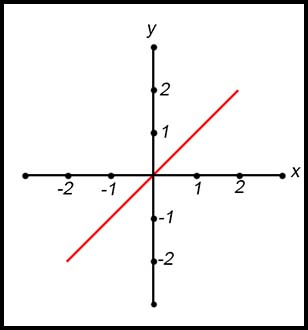The line shown in the graph above passes through the origin and the pints (1, 1), (2, 2), etc. Hence the gradient is 1 and there is no intercept. Hence the equation is y = x. 7.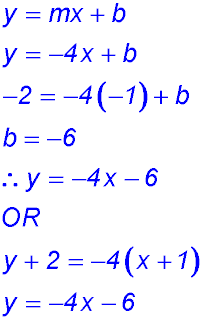8.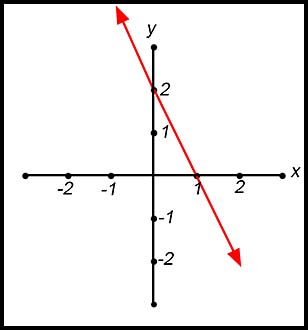The rise is 2 and the run is 1 and the slope is negative. Hence the gradient is -2. The y intercept is 2.9. The gradient of 0 indicates the line is horizontal. As the line passes through the point (1, -2), the y value is constant. Hence the equation is y = -2. Given two points (answer in general form). 10. Determine the equation of the line shown in the graph below: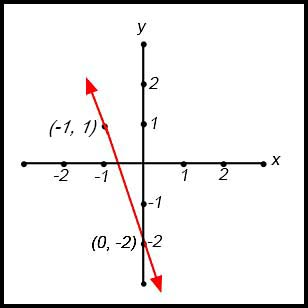First determine the gradient between the indicated points. The rise is 3 and the run is 1 and the line slopes down. So the gradient is -3.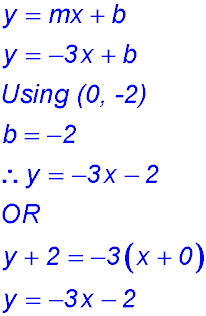11. The line through the points (10, 3) and (15, 8) has a gradient of +1 (draw a quick sketch).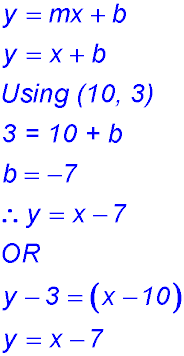12. Quickly sketch the line through the points (-12, 3) and (4, -5). The rise is 8 and the run is 16 and the line slopes down. Hence the gradient is -0.5.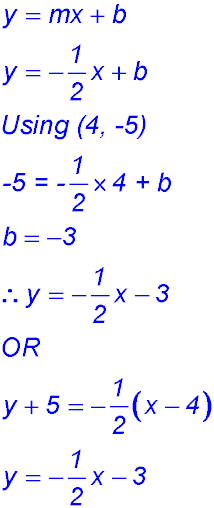13. Quickly sketch the line through the points (3.5, 2.7) and (5.5, 10.7). Rise is 8 and run is 2 - so gradient = 4.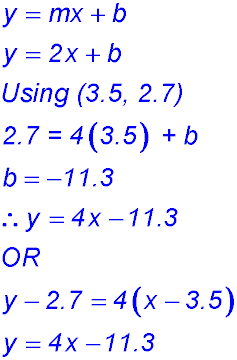14. The line through the points (3, -2) and (3, 2) is vertical because the x values are the same. There is no run. Hence the equation is x = 3. Given data in a table. 15. (i) As x goes from 4 to 5 (an increase of 1), y decreases from 7 to 3 (a fall of 4). So the gradient is -4. The equation is y - 7 = -4(x - 4). ∴ y = -4x + 23 (ii) When y = -33 -33 = -4x + 23 4x = 56 x = 14. 16. (i) For the y intercept, x = 0. Hence y = -1. As x goes from 0 to 1, y increases from -1 to +1 (a rise of 2). So the gradient is 2. The equation is y = 2x - 1. Given data in a diagram. 17. From the diagram, the y intercept is 6 and the gradient is 6/3 = 2 but negative as the line slopes down. Hence the equation is y = -2x - 6 18. From the diagram, the y intercept is -3 and the gradient is 3/9 = 1/3 and positive as the line slopes up. Hence the equation is y = x/3 -3 or x - 3y - 9 = 0. Given a geometrical context. 19. The line is horizontal so all y values are the same. Hence the equation is y = 15. 20. The line is vertical so all x values are the same. Hence the equation is x = 26. 17. Draw a quick sketch. Mark in A (2, 3). The line AC is 3x - y - 9 = 0 and it cuts the y axis at -9 which is C. Now draw in the rectangle ABCD. B is horizontal from A and D is horizontal from C: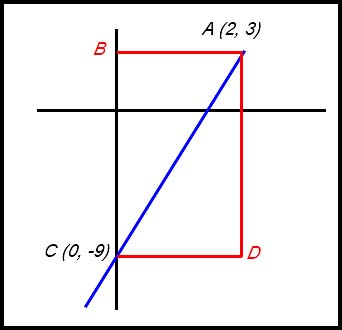Hence B is (0, 3) and D is (2, -9). 18.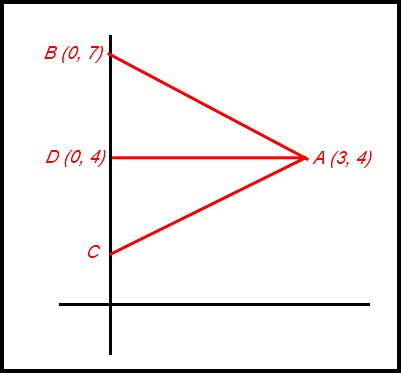AD is the line from the vertex A perpendicular to the base (i.e. the y axis). It has coordinates (0, 4). Note: AD bisects the base - a basic property of an isosceles triangle. The distance DB is 3 units so DC must also be 3 units. Hence C has coordinates (0, 1). Applications to the physical world. 19. (i) Ish begins her saving with a deposit of \$500 at time = 0. Hence that is the value to be marked on the vertical (investment) axis. Ish saves at \$40 per week which is the gradient of her investment. Hence we have: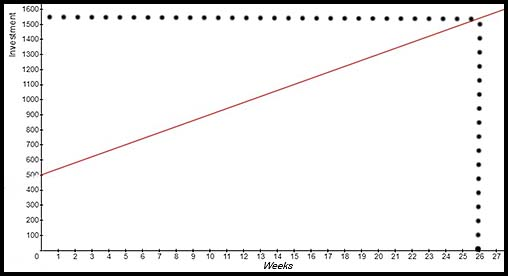(ii) The equation to express her investment strategy mathematically is Investment = \$500 + \$40t (iii) Using this equation for t = 26, Ish will have saved \$500 + \$40(26) = \$1,540 20. (i) The car is stationary initially - so at t = 0, speed = 0. Hence the intercept on the vertical (speed) axis is 0. The car increases its speed at a constant rate of 0.4 m/sec - and so this value is used for the gradient of the line.(ii) Given the above information and the basic format of the line as y = mx + b, we write the required equation linking the two variables as Speed = 0.4 × seconds (iii) Substituting into this equation: 16.7 = 0.4×Secs Hence 41.75 seconds. The graph shows the line coming from 16.7 m/sec on the vertical axis to the line and then down to nearly 42 seconds. 21. (i) 6×8 + 79 = 127 cm. (ii) The gradient in a linear equation is the coefficient of the independent variable. Here that variable is AGE and the coefficient (i.e. the gradient) is 6. The value of 6 indicates that for every increase in age of 1 year (in the given age range), the height jumped by a girl is expected to increase by 6 cm. (iii) The linear equation is based (i.e. developed) on elated to girls aged from 6 to 11 years. Beyond that age range, the gradient (increase in height jumped) might not be 6 cm. The relationship would almost certainly be different. Hence a different equation would have to be developed for other age ranges - and it might not even be linear.